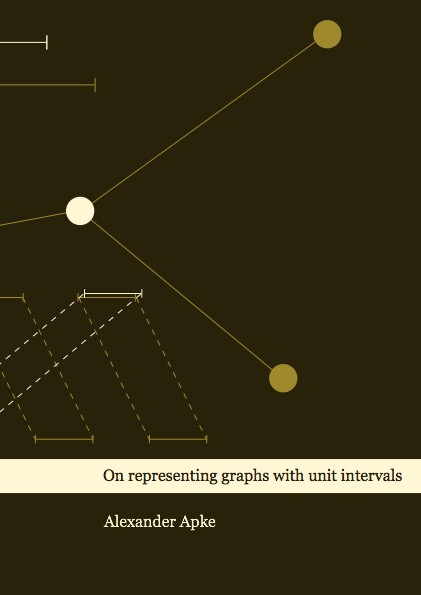Datenbestand vom 13. Juni 2019WICHTIGER HINWEIS
DER VERLAG IST IN DER ZEIT VOM 12.06.2019 BIS 23.06.2019 AUSCHLIESSLICH PER EMAIL ERREICHBAR.

aktualisiert am 13. Juni 2019

ISBN 9783843937306Euro 60,00 inkl. 7% MwSt

978-3-8439-3730-6, Reihe Informatik

Alexander Apke
On representing graphs with unit intervals

111 Seiten, Dissertation Universität Köln (2018), Hardcover, A5

Zusammenfassung / Abstract

In this thesis, we firstly introduce the non-unit count as a parameter of an interval graph or an interval order. The non-unit count is the smallest number of intervals whose lengths have to deviate from unit length in an assigned interval representation.

We characterize those interval graphs that have non-unit count one. Further, we investigate the special case where intervals are forced to have at least unit length. In that case, we call the minimal number of deviating intervals the normalized non-unit count and we show that this number equals the number of centers of the graph.

In contrast to the non-unit count, it turns out that both the normalized non-unit count and the property of being a partial order with non-unit count one are comparability invariants.

In the subsequent chapters, we focus on the semiorder dimension of a partial order, another parameter to measure a partial orders' distance to being a semiorder.

Habib et al. have shown that interval dimension is a comparability invariant. Felsner and Möhring could prove this for semiorder dimension two. We examine the question whether this carries over to semiorder dimension 3. We introduce the excess representation as a specific trapezoid representation. For partial orders of semiorder dimension three, we show that admitting such a representation is a comparability invariant. We conjecture that every partial order of semiorder dimension three admits an excess representation. This would imply that semiorder dimension three is a comparability invariant as well. We prove the conjecture for partial orders induced by a specific graph class.

The semiorder dimension of a partial order may be arbitrarily large. The same is true for the semiorder dimension of an interval order, as shown by Fishburn. For partial orders of a fixed semiorder dimension greater than 1, no characterization is known.

We give a characterization for the class of interval orders with semiorder dimension two as well as for the class of graphs that induce these orders. Our characterizations admit efficient recognition algorithms. Further, we provide a sufficient condition that applies for all partial orders of semiorder dimension two.